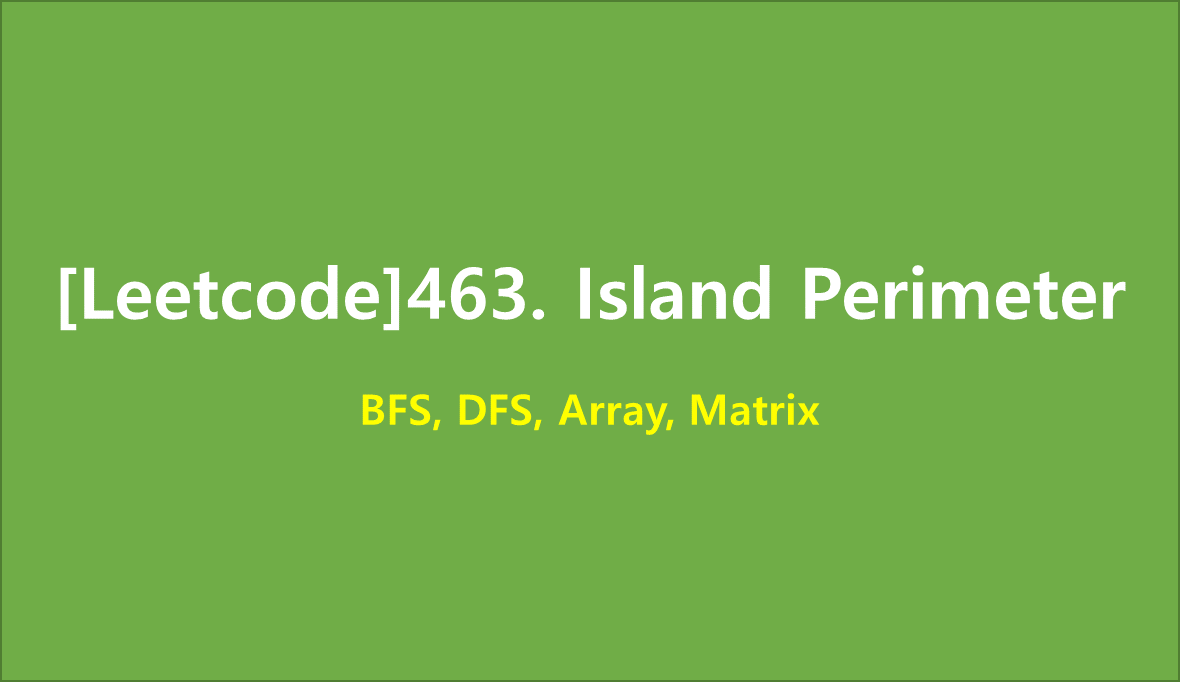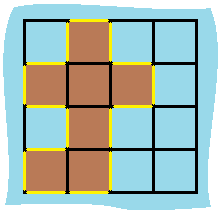# [Leetcode]463. Island Perimeter

limelimejiwon·2022년 5월 18일
0

## Python으로 공부하는 코딩테스트

목록 보기
50/67## 📄 Description

You are given row x col grid representing a map where grid[i][j] = 1 represents land and grid[i][j] = 0 represents water.

Grid cells are connected horizontally/vertically (not diagonally). The grid is completely surrounded by water, and there is exactly one island (i.e., one or more connected land cells).

The island doesn't have "lakes", meaning the water inside isn't connected to the water around the island. One cell is a square with side length 1. The grid is rectangular, width and height don't exceed 100. Determine the perimeter of the island.

### Example 1:Input: grid = [[0,1,0,0],[1,1,1,0],[0,1,0,0],[1,1,0,0]]
Output: 16
Explanation: The perimeter is the 16 yellow stripes in the image above.

### Example 2:

Input: grid = []
Output: 4

### Example 3:

Input: grid = [[1,0]]
Output: 4

### 🚩 Constraints:

• row == grid.length
• col == grid[i].length
• 1 <= row, col <= 100
• grid[i][j] is 0 or 1.
• There is exactly one island in grid.

## 💻 My Submission

class Solution:
cnt=0
def islandPerimeter(self, grid: List[List[int]]) -> int:
m, n=len(grid), len(grid)

def dfs(i,j,grid):
if not grid[i][j]:
return

grid[i][j]='#'
if ni<0 or nj<0 or ni>=m or nj>=n:
self.cnt+=1
continue
if grid[ni][nj]==0:
self.cnt+=1
elif grid[ni][nj]==1:
dfs(ni, nj, grid)

for i in range(m):
for j in range(n):
if grid[i][j]==1:
dfs(i,j,grid)
return self.cnt

## Clean Code

class Solution(object):
def islandPerimeter(self, grid):
"""
:type grid: List[List[int]]
:rtype: int
"""
if not grid:
return 0

adjacent = (i + 1, j), (i - 1, j), (i, j + 1), (i, j - 1)
res = 0
if x < 0 or y < 0 or x == len(grid) or y == len(grid) or grid[x][y] == 0:
res += 1
return res

count = 0
for i in range(len(grid)):
for j in range(len(grid)):
if grid[i][j] == 1:
return count